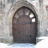Choose Language Hide Translation Bar
Highlighted

## Multiple Comparison -- Minimum Significant Difference (LSD or HSD)

Does anyone know if JMP will give you MSD value (minimum significant difference) for a multiple comparison test (i.e. Tukey's HSD)? If so please let me know how to get it. THANKS!

1 ACCEPTED SOLUTION

Accepted Solutions
Highlightedsusan_walsh1
Staff (Retired)

## Re: Multiple Comparison -- Minimum Significant Difference (LSD or HSD)

The minimum significant difference is not the same for all pairwise comparisons unless the sample sizes for all groups are equal. When sample sizes are not equal, then the minimum significant difference will vary from comparison to comparison.

If you are using the Fit Y by X platform (Oneway Analysis), you can determine the minimum significant difference as described earlier. When you do the comparisons, you will get an LSD or HSD Threshold Matrix which is the absolute value fo the difference minus the LSD or HSD. From the red triangle menu, you can obtain the Difference Matrix. You can use these together to determine the LSD or HSD.

In the Fit Model platform, you will get a matrix of the LSMeans Differences for the multiple comparison method chosen. In that case the test statistic (Q for Tukey or t for Student's t) is shown above the matrix and the standard error of the difference is shown in each cell of the matrix. If you multiply the test statistic by the standard error of the difference, you obtain the minimum significant difference (LSD or HSD).

5 REPLIES 5
Highlighted

## Re: Multiple Comparison -- MSD

It's called LSD (least significant distance).
When you are doing multiple comparison test, you get the table called
Abs(Dif) - LSD
To get the LSD value subtract the value from the table above from the difference between the means found in the difference matrix in the same window.

Hope this helps...
Highlighted

## Re: Multiple Comparison -- MSD

When I separate means using Tukey's HSD, I get a LSMeans Differences Tukey HSD Table. Is there not a simply way to get JMP to generate the LSD value? I ask because I still don't follow what to subtract from what, and I deal with multi-factorial ANOVAs, so I can't do it all by hand.

Thanks
Highlighted

## Re: Multiple Comparison -- MSD

After you've run your "Fit Model" dialog, go to the "Effect Details" tab, and expand that. You'll find there a tab for each of the factors you've included in the model, each of which has a red triangle on the tab. Click on that red triangle, and you'll get a menu from which you can select either "LSMeans Student's t" or "LSMeans Tukey HSD". If you do that you'll get two tables: the first one shows the SED, lower confidence limit and upper confidence limits (from which you can calculate the LSD) of each pairwise comparison between every level of your factor, and the second one gives you a "letters chart" in which all the means are ranked from largest to smallest, with columns of letters joining all groups of levels that are not significantly different at whatever significance level you wanted. The letters chart gives you an immediate feel for what's significantly different from what, and I find is also a very convenient summary table for including in reports, not least because you can right-click on it to create a data table which you can then export directly as an Excel spreadsheet.

The cutoff level is normally alpha=0.05, but you can change that - see this thread for details: http://support.sas.com/forums/thread.jspa?messageID=29987産
Highlighted

## Re: Multiple Comparison -- MSD

Thanks.

I follow expanding the tabs, but I am still confused:

1. From the LSMeans Student's T tab, how is SED calculated from the LSmeans below the matrix shown?

2. Once the SED is calculated, how do you calculate LSD?

I often use the letters chart, but if I have many response variables I get an alphabet soup of information that can be unwieldy in a paper, which is why I sometimes just prefer to include an LSD value.

I guess I am still surprised that JMP doesn't just have a function to give you an immediate value for SED and LSD when you have many response variables.

Many thanks!
Highlightedsusan_walsh1
Staff (Retired)

## Re: Multiple Comparison -- Minimum Significant Difference (LSD or HSD)

The minimum significant difference is not the same for all pairwise comparisons unless the sample sizes for all groups are equal. When sample sizes are not equal, then the minimum significant difference will vary from comparison to comparison.

If you are using the Fit Y by X platform (Oneway Analysis), you can determine the minimum significant difference as described earlier. When you do the comparisons, you will get an LSD or HSD Threshold Matrix which is the absolute value fo the difference minus the LSD or HSD. From the red triangle menu, you can obtain the Difference Matrix. You can use these together to determine the LSD or HSD.

In the Fit Model platform, you will get a matrix of the LSMeans Differences for the multiple comparison method chosen. In that case the test statistic (Q for Tukey or t for Student's t) is shown above the matrix and the standard error of the difference is shown in each cell of the matrix. If you multiply the test statistic by the standard error of the difference, you obtain the minimum significant difference (LSD or HSD).

Article Labels

There are no labels assigned to this post.